электронная
60
печатная A5
333
12+# Бесплатный фрагмент - Review. Benzene on the basis of the three-electron bond

#### Theory of three-electron bond in the four works with brief comments (review). 2016.

Объем:
132 стр.
Возрастное ограничение:
12+
ISBN:
978-5-4483-6946-9
электронная
от 60
печатная A5
от 333

I express my deep gratitude to my son, Bezverkhniy Vitaliy Volodymyrovich, for participation in the development of the theory (some parts as a co-author), and for his invaluable contribution to the English translation.

Abstract: Using the concept of three-electron bond we can represent the actual electron structure of benzene and other molecules, explain specificity of the aromatic bond and calculate the delocalization energy. Gives theoretical justification and experimental confirmation of existence of the three-electron bond.

It was shown, that functional relation y = a + b/x + c/xfully describes dependence of energy and multiplicity of chemical bond from bond distance.

Keywords: benzene, three-electron bond, semi-virtual particle, fermion, entangled quantum state, Interfering Universe.

1. Introduction

Chemical bond has been always a basis of chemistry. Advancement of chemical science can be considered as evolution, development of concepts about chemical bond. Aromatic bond is fundamental basis of organic chemistry. Concept of three-electron bond in benzene molecule enable to explain specificity of aromatic bond. It also becomes apparent, why planar molecules with 6, 10 etc. electrons (according to Hückel rule 4n +2) must be aromatic, and planar molecules with 4, 8 etc. electrons cannot be aromatic by definition.

Description of chemical bond, that is given by quantum theory, especially in terms of method of molecular orbitals, is just a mathematical model. This model is an approximate representation of molecules and its bonds, whereas quantum-mechanical calculations of organic molecules require considerable simplifications and are extremely complicated.

Concept of three-electron bond and developed mathematical relations in this work are rather simple, illustrative and give exact results of different values (bond multiplicity, chemical bound energy, delocalization energy of benzene). One must clearly imagine, that three-electron bond is joint interaction of three electrons with relative spins, that results in new type of chemical bond (A • • • A (+ — +), A • • • B (+ — +)). This bond type, three-electron bond, makes possible to describe real molecules of organic and inorganic compounds without invoking virtual structures, which do not exist in real terms.

Using of three-electron bond before description of benzene molecule enables to determine delocalization energy of benzene in an elementary way, understand why multiplicity of С-С bond of benzene is more than 1.5 and to understand the main point of aromatic bond in general, which is appeared to be rather illustrative. Besides, for determination of delocalization energy it is not required to select reference structures. Delocalization energy follows from the concept of aromaticity of benzene and its structure on the basis of three-electron bond.

I note that the three-electron bond to describe the benzene molecule used W.O. Kermak, R. Robinson and J. J. Thomson at the beginning of the 20th century [5, 6].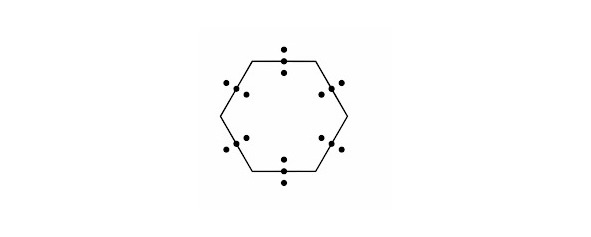Benzene molecule with three-electron bond (W.O. Kermak and R. Robinson, J. J. Thomson).

But since it is not taken into account the spin of electrons, have already started cyclooctatetraene problems and therefore the description of the benzene molecule by a three-electron proved unsuccessful. Using the three-electron bond with multiplicity of 1.5 and take account of the spin of each electron leads to very good results in the description of the benzene molecule and explain the aromaticity in general. With the help of three-electron bond with multiplicity of 1.5 can be represented by a real formula of many organic and inorganic molecules without the aid of virtual structures.

2. Structure of the benzene molecule on the basis of the three-electron bond

2.1. Results and discussion

Supposing that the chemical bond between two atoms can be established by means of three electrons with oppositely oriented spins (↑↓↑) the structure of the benzene molecule can be expressed as follows (see figure 1 and figure 2):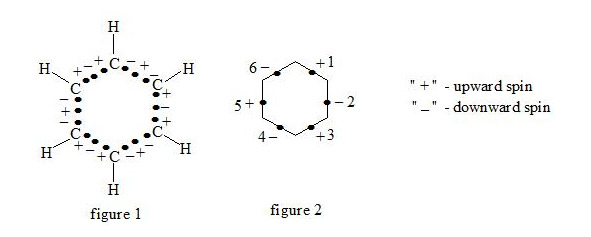benzene molecule on the basis of the three-electron bond, spin

It is interesting to point out that spins of central electrons on opposite sides have an opposite orientation (see figure 2). Now let us consider in detail the interaction of six central electrons between themselves. They will be itemized as shown in figure 2. As the spin of electron 1 and those of electrons 2 and 6 are oppositely oriented (see figure 2) (1 (+), 2 (-), 6 (-)), electron 1 will be attracted to electrons 2 and 6 respectively. Let’s indicate that the distance between electrons 1 and 6 or 1 and 2 is equal to 1.210 Å which can be easily shown taking into account the distance between atoms of carbon in benzene to be 1.397 Å and the angle between carbon atoms amount to 120 degrees. Let us compare the distance between electrons 1 and 6 and 1 and 2 bond lengths in ethane, ethylene and acetylene :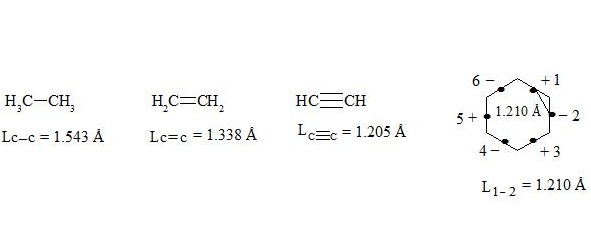bond lengths in ethane, ethylene, acetylene and distance between electrons (1 and 2) in benzene

As we observe, the distance between central electrons 1 and 2 and 1 and 6 of the benzene molecule is approximately equal to that between carbon atoms in the acetylene molecule, therefore, the interaction between electrons 1 (+) and 2 (-) and 1 (+) and 6 (-) has to be rather considerable. Let us express the attraction with arrows. According to summing up vectors the resultant vector will be directed to the centre, which means that electron 1 under the influence of electrons 2 and 6 will move to the centre (figure 3):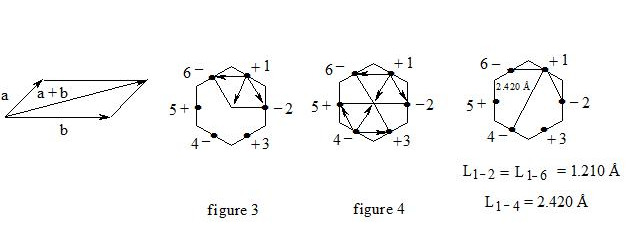benzene on the basis of the three-electron bond, summing up vectors

If we take a look at electron 4 we see the similar situation with it (figure 4) and it will also move to the centre and, more importantly, its spin and that of electron 1 will be oppositely oriented, i.e. electron 1 (+) and electron 4 (-) will be attracted through the cycle. Electrons 6 (-) and 3 (+) and electrons 2 (-) and 5 (+) will interact similarly. The distance between electrons 1 and 4 in benzene is equal to 2.420 Å. It is interesting, that this distance is twice as much than distance between electrons 1 and 2, or between electrons 1 and 6 (1.210 Å ∙ 2 = 2.420 Å). This interaction through the cycle constitutes the essence of the delocalization of electrons, of course together with a three-electron bond. Since besides the three-electron bond in the benzene molecule there is an interaction through the cycle, meaning that the benzene nucleus undergoes a kind of compression it is clear that the c-c bond multiplicity in benzene will exceed 1.5.

So, the aromatic system is a cyclic system with three-electron bonds where an interaction of central electrons through the cycle is observed. In the benzene molecule there are three interactions through the cycle-pairwise between electrons 1 (+) and 4 (-), 2 (-) and 5 (+), 3 (+) and 6 (-), as shown in figure 5: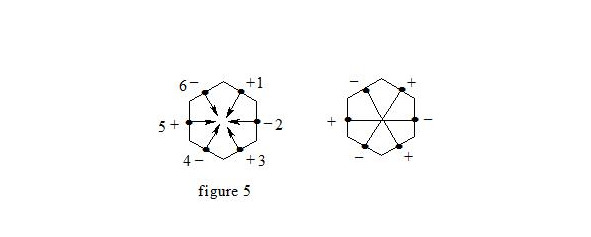benzene on the basis of the three-electron bond, interaction through the cycle

Carbon atoms in benzene are sp²-hybridized. The three-electron bond between carbon atoms in the benzene molecule can be represented as follows:

Carbon atoms in benzene have an octet equal to 8 (3 +3 +2 = 8). It should be pointed out that due to the largest distance from the atoms nuclei the central electrons of the three-electron bond are supposed to be the most mobile compared to other electrons of the three-electron bond. The interaction of central electrons with opposite spins through the cycle can easily explain why cyclobutadiene and cyclooctatetraene are not aromatic compounds: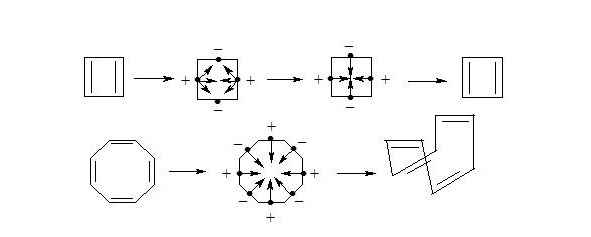cyclobutadiene and cyclooctatetraene (three-electron bond)

As we see both in cyclobutadiene and cyclooctatetraene, electrons interacting through the cycle have the same spins and, clearly, will be repulsed, therefore there will be no interaction through the cycle and the molecule will not be aromatic. In cyclobutadiene at the expense of small distance it causes the appearance of antiaromatic properties, and in cyclooctatetraene there is a possibility of formation of non-planar molecule, where interaction of central electrons becomes impossible and molecule losing the interaction through the cycle loses also three-electron bonds, that results in a structure, in which single and double bonds alternate.

Explanation, that cyclooctatetraene is non-aromatic, because it is non-planar and does not hold water, insomuch as dianion of cyclooctatetraene is aromatic and has planar structure , .Planar

X-ray crystal structure analysis determined crystal structure of potassium salt of dianion 1,3,5,7-tetramethylcyclooctatetraene , .

Octatomic cycle is planar with lengths of С-С bonds nearly 1.41 Å.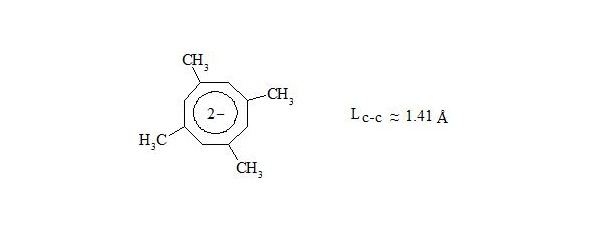Planar

From the mentioned above we can make a conclusion: cyclooctatetraene conforms to the shape of bath tub not because of high angular pressure (15°) at planar structure, but because by interaction through the cycle central electrons of three-electron bonds have equal spin and will push away. Thus for energy reduction cyclooctatetraene conforms to the shape of bath tub and becomes non-planar, that disables interaction of central electrons.

Cyclobutadiene represents rectangular high reactivity diene [8, p. 79].

It is also interesting to observe cyclodecapentaene (cis-isomer  -annulene).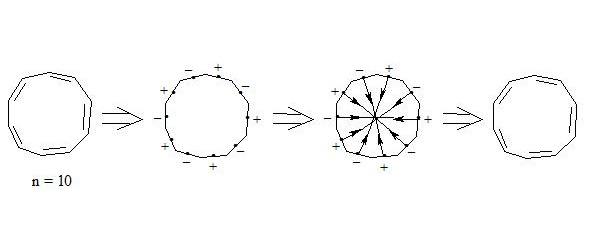cyclodecapentaene (three-electron bond)

Бесплатный фрагмент закончился.
Купите книгу, чтобы продолжить чтение.
электронная
от 60
печатная A5
от 333